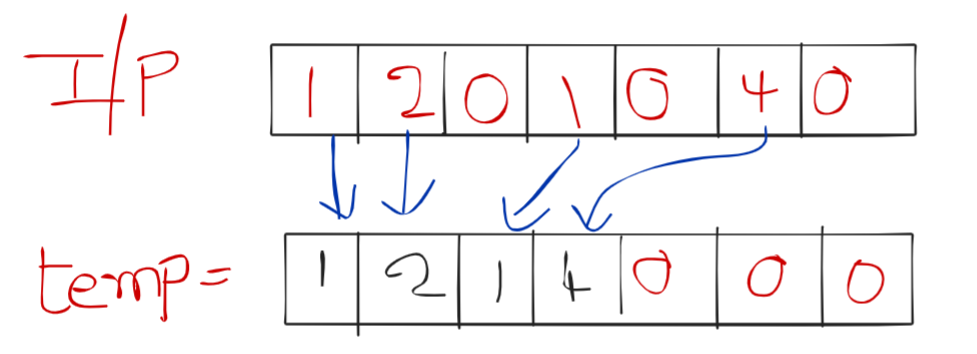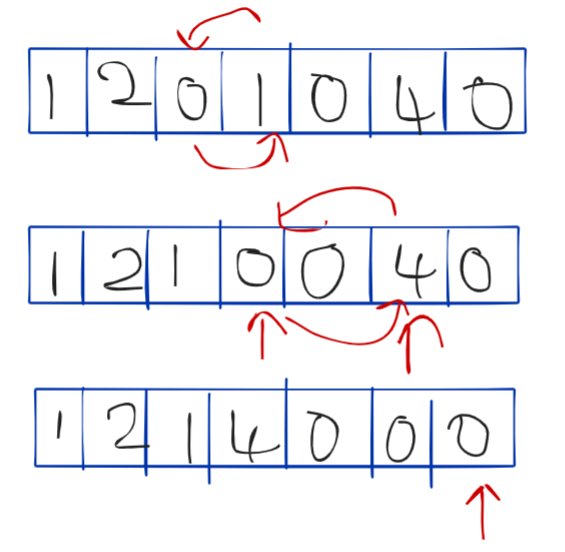# Move all Zeros to the end of the array

In this article we will learn how to solve the most asked coding interview problem: “Move all Zeros to the end of the array”

Problem Statement: You are given an array of integers, your task is to move all the zeros in the array to the end of the array and move non-negative integers to the front by maintaining their order.

Examples:

```Example 1:
Input: 1 ,0 ,2 ,3 ,0 ,4 ,0 ,1
Output: 1 ,2 ,3 ,4 ,1 ,0 ,0 ,0
Explanation: All the zeros are moved to the end and non-negative integers are moved to front by maintaining order

Example 2:
Input: 1,2,0,1,0,4,0
Output: 1,2,1,4,0,0,0
Explanation: All the zeros are moved to the end and non-negative integers are moved to front by maintaining order```

### Solution

DisclaimerDon’t jump directly to the solution, try it out yourself first.

## Solution 1:Brute Force

Approach and thought process:

Here, our 1st task is to put non-negative elements in the front of the array, So we can create a new temporary array and update indices of this temp array from starting with non-negative elements and while doing this we can count the number of Zeros also, So we will count the number of zeros and fill remaining indices of temp array with zero.

Let’s see the algorithm:

• Create a temp array of length input array
• Traverse through array and if a non negative elements encounter then put this element in the temp array at zero index and increment index
• Fill the zeros in remaining places of temp arrayCode:

## C++ Code

``````#include<bits/stdc++.h>
using namespace std;

void zerosToEnd(int arr[],int n) {

int temp[n];
int k=0;
for (int i=0;i<n;i++){
if (arr[i]!=0){
temp[k]=arr[i];
k++;
}
}

while (k<n){
temp[k]=0;
k++;
}
for(int i=0;i<n;i++)
{
cout<<temp[i]<<" ";
}
}

int main() {
int arr[] ={ 1,2,0,1,0,4,0};
zerosToEnd(arr,7);
}

``````

Output: 1 2 1 4 0 0 0

Time complexity: o(n)

Space complexity: o(n)

## Java Code

``````public class MoveZeros{
public static void main(String[] args) {
int arr[] ={ 1,2,0,1,0,4,0};
zerosToEnd(arr);
}
public static void zerosToEnd(int[] arr) {

int n = arr.length;
int temp[] = new int[n];
int k=0;
for (int i=0;i<n;i++){
if (arr[i]!=0){
temp[k]=arr[i];
k++;
}
}

while (k<n){
temp[k]=0;
k++;
}
for(int i=0;i<n;i++)
{
System.out.print(temp[i]+" ");
}
}
}
``````

Output: 1 2 1 4 0 0 0

Time complexity: o(n)

Space complexity: o(n)

## Solution 2:Optimal Approach

If we find a zero (say its index is 2) while traversing that means this place should be occupied by the immediate next non zero elements (so that order will be maintained for non zero elements) so we will place a pointer here (at 2) and we will try to find next non-negative integer using another pointer, once it was found that means zero places (2nd index) has to be occupied by this non-negative number and non-negative number will be occupied by zero.

Algorithm:

1. Start traversing from the first occurrence index of Zero
2. Tak 2 variables (i,j), i will be at the first occurrence of zero and j is at i+1
3.  If element at j index is not zero then swap elements at i,j and increment i,j
4. If the element at j index is zero then only increment j.Code:

## C++ Code

``````#include<bits/stdc++.h>

using namespace std;
void zerosToEnd(int arr[], int n) {

//finding first zero occurrence
int k = 0;
while (k < n) {
if (arr[k] == 0) {

break;
} else {
k = k + 1;
}
}

//finding zeros and immediate non-zero elements and swapping them
int i = k, j = k + 1;

while (i < n && j < n) {
if (arr[j] != 0) {
int temp = arr[i];
arr[i] = arr[j];
arr[j] = temp;
i++;

}

j++;

}
for (i = 0; i < n; i++) {
cout << arr[i] << " ";
}
}

int main() {
int arr[] ={ 1,2,0,1,0,4,0};
zerosToEnd(arr, 7);
}``````

Output: 1 2 1 4 0 0 0

Time complexity: O(n)

Space complexity: O(1)

## Java Code

``````public class MoveZeros {
public static void main(String[] args) {
int arr[] ={ 1,2,0,1,0,4,0};
zerosToEnd(arr);

}
public static void zerosToEnd(int[] arr) {

//finding first zero occurrence
int k = 0;
while (k < arr.length) {
if (arr[k] == 0) {

break;
} else {
k = k + 1;
}
}

//finding zeros and immediate non-zero elements and swapping them
int i = k, j = k + 1;

while (i < arr.length && j < arr.length) {
if (arr[j] != 0) {
int temp = arr[i];
arr[i] = arr[j];
arr[j] = temp;
i++;

}

j++;

}
for (i = 0; i < arr.length; i++) {
System.out.print(arr[i] + " ");
}
}
}``````

Output: 1 2 1 4 0 0 0

Time complexity: O(n)

Space complexity: O(1)

Special thanks to Sai bargav Nellepalli for contributing to this article on takeUforward. If you also wish to share your knowledge with the takeUforward fam, please check out this article